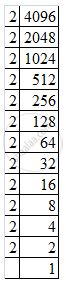Share

# Find the Square Root the Following by Prime Factorization. 4096 - CBSE Class 8 - Mathematics

ConceptSquare Roots - Finding Square Root Through Prime Factorisation

#### Question

Find the square root  the following by prime factorization.

4096

#### Solution

Resolving 4096 into prime factors:
4096 = 2 x 2 x 2 x 2 x 2 x 2 x 2 x 2 x 2 x 2 x 2 x 2Grouping the factors into pairs of equal factors:
4096 = (2 x 2) x (2 x 2) x (2 x 2) x (2 x 2) x (2 x 2) x (2 x 2)
Taking one factor for each pair, we get the square root of 4096:
(2 x 2) x (2 x 2) x (2 x 2) = 64

Is there an error in this question or solution?

#### APPEARS IN

Solution Find the Square Root the Following by Prime Factorization. 4096 Concept: Square Roots - Finding Square Root Through Prime Factorisation.
S